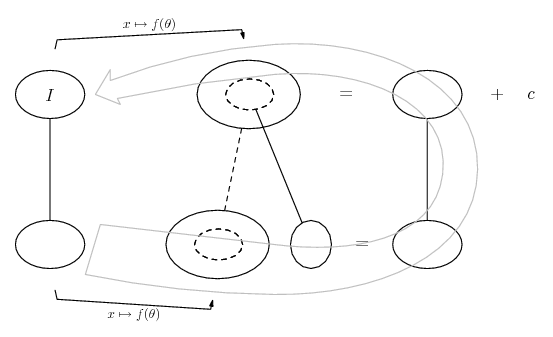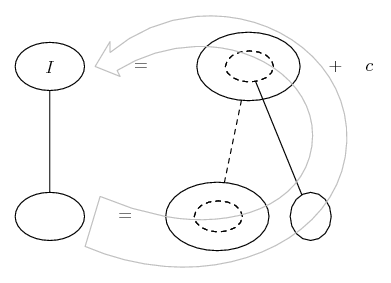# Thread: Evaluate the Integral

1. ## Evaluate the Integral

This one has had me stuck for a good hr.

The integral has a min of 0 and a max of 1.

S (4-x)/(sqrt(4-x^2)) dx

I'm trying to use the formula S (du)/(sqrt(a^2-u^2)) = arcsin u/a + C

I set "u" = (x^2), "du" = 2x, and a = 2.

Typically the problems I get will have the du equal the numerator and it's pretty easy from there. But my du does not equal the numerator and I'm stuck.

I think the answer for the interval (disregarding the min and max) is:

Sqrt(4-x^2) +
4 arcsin(x/2) + C.

And the part in underlined bold I have absolutely no idea where it came from.

And the final answer:
-2 + Sqrt(3) + (2pi)/3

Basically I cannot figure a way to get that answer or the steps involved. Can anyone help? Thank you.

2. It is easy to obeserve that is...

$\displaystyle \displaystyle \frac{4-x}{\sqrt{4-x^{2}}}= \frac{2}{\sqrt{1-(\frac{x}{2})^{2}}} - \frac{\frac{x}{2}}{\sqrt{1-(\frac{x}{2})^{2}}}$

Kind regards

$\displaystyle \chi$ $\displaystyle \sigma$

3. $\displaystyle \displaystyle \int\frac{4-x}{\sqrt{4-x^{2}}}\;{dx} = \int \frac{4}{\sqrt{4-x^2}}-\frac{x}{\sqrt{4-x^2}}\;{dx}= 4\int\frac{1}{\sqrt{1-x^2}}\;{dx}+\int\frac{(1-x^2)'}{2\sqrt{1-x^2}}\;{dx}.$

The first one should be easy enough. The second is of the form $\displaystyle \int\frac{f'(x)}{2\sqrt{f(x)}}\;{dx} = \sqrt{f(x)}+k$.

4. I am still not following.

@Chisigma. I see that you broke them up to two separate integrals and divided by 2. But after that I don't see where to go from there.

@TheCoffeeMachine. I see what you did on the first two steps but on the 3rd one when you add them, I am lost. In the front of my calc book I have a sheet with basic integration formulas, it doesn't show anything that relates to yours. I also don't understand where you got the second form. Is their a good site with more formulas?

I also tried to look at how Wolfram Alpha solved it

&#40;4-x&#41;&#47;&#40;sqrt&#40;4-x&#94;2&#41;&#41; - Wolfram|Alpha

And the part that me throws me off on their solution is that they set x = 2sin(u) and u = arcsin (x/2)

I got a good 2+hrs into this one problem and 3 pages of scratch paper. I'm at a loss. Thank you in advance!

Derek Z

5.Originally Posted by DerekZ10I am still not following.

@Chisigma. I see that you broke them up to two separate integrals and divided by 2. But after that I don't see where to go from there.
Just in case a picture helps...... where (key in spoiler) ...

Spoiler:... is the chain rule. Straight continuous lines differentiate downwards (integrate up) with respect to the main variable (in this at first case x, then after the 'sub', theta), and the straight dashed line similarly but with respect to the dashed balloon expression (the inner function of the composite which is subject to the chain rule).

The general drift is...Then back substitute from sin theta to x/2 to find F.

What TCM was hinting towards is kind of like this...... where (key in spoiler) ...

Spoiler:
The general drift is...Edit: BTW, using the trig sub for the whole fraction like Wolfram does...... means that after back-substituting you have an expression

$\displaystyle 2 \cos(\arcsin(\frac{x}{2}))$

which with some Pythag will iron out to the same as above.

________________________________________________

Don't integrate - balloontegrate!

Balloon Calculus; standard integrals, derivatives and methods

Balloon Calculus Drawing with LaTeX and Asymptote!

6.Originally Posted by DerekZ10∫ (4-x)/(sqrt(4-x^2)) dx

I'm trying to use the formula S (du)/(sqrt(a^2-u^2)) = arcsin u/a + C
...

I think the answer for the integral (disregarding the min and max) is:

Sqrt(4-x^2) +
4 arcsin(x/2) + C.

And the part in underlined bold I have absolutely no idea where it came from.
...

Basically I cannot figure a way to get that answer or the steps involved. Can anyone help? Thank you.
Two others have covered this quite well. I'll also give it a try.

$\displaystyle \displaystyle {{4-x}\over{\sqrt{4-x^2}}}={{4}\over{\sqrt{4-x^2}}}+{{-x}\over{\sqrt{4-x^2}}}$

You apparently have no problem with the integral: $\displaystyle \displaystyle \int {{4}\over{\sqrt{4-x^2}}} dx\,.$

For the integral $\displaystyle \displaystyle \int {{-x}\over{\sqrt{4-x^2}}} dx\,,$ use the substitution:

$\displaystyle \displaystyle u = 4-x^2\quad\to\quad du=-2x\,dx\,.$

Can you take it from here?

7. Yes I got it, thank you!

#### Search Tags

evaluate, integral